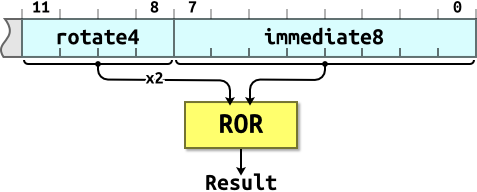# ARM > Introduction to ARM > Immediate Values

by on

## Immediate Values

• You can’t fit an arbitrary 32-bit value into a 32-bit instruction word.
• ARM data processing instructions have 12 bits of space for values in their instruction word. This is arranged as a four-bit rotate value and an eight-bit immediate value:The 4-bit rotate value stored in bits 11-8 is multiplied by two giving a range of 0-30 in steps of two.

Using this scheme we can express immediate constants such as:

• `0x000000FF`
• `0x00000FF0`
• `0xFF000000`
• `0xF000000F`

But immediate constants such as:

• `0x000001FE`
• `0xF000F000`
• `0x55550000`

…are not possible.

An assembler will convert big values to the rotated form. Impossible values will cause an error.

Some assemblers will use other tricks such as using `MVN` instead of `MOV` to form the bitwise complement of the required constant. For example the impossible instruction `MOV r0,#0xFFFFFFFF` could be assembled as `MVN r0,#0`.

## Remarks

The impact of this is that some constants are “ARM friendly”. Some are not. Study of the numbers you’re using can sometimes reveal scope for optimisation.

You can form constants wider than those available in a single instruction by using a sequence of instructions to build up the constant. For example:

```MOV r2, #0x55           ; R2 = 0x00000055
ORR r2, r2, r2, LSL #8  ; R2 = 0x00005555
ORR r2, r2, r2, LSL #16 ; R2 = 0x55555555
```

…or load the value from memory:

```LDR r2, =0x55555555
```

The pseudo-instruction `LDR Rx,=const` tries to form the constant in a single instruction, if possible, otherwise it will generate an `LDR`.